# Introduction

This article covers one method of attaching wires to a bare lithium-polymer battery pouch. This could also be done using a battery spot welder, clamping, or screwing.

Warning: Lithium Ion and Lithium Polymer batteries can catch on fire if shorted, punctured or overheated.
Be careful when soldering that the battery does not become hot.
Ensure you have an appropriate fire extinguisher nearby.

# Step 1: Wire Prep

Before you solder the terminals, plan your wiring.
Where should the battery wires exit the battery? In what direction will they go, and how far? (A scale printout of the layout may help)

Strip and tin the wire ends.
Each bare end should be slightly shorter than the battery tab’s width.

# Step 2: Tab Prep

Peel back one of the battery terminals.

With a small file, roughen the outer half of the battery tab.

Fold the end of the battery tab over, to create a small ‘hook’.
It should be just large enough to fit the wire in.

Put a tiny drop of flux in the hook.
You can spread it around with a piece of wire.

# Step 3: Soldering

Place the wire into the hook – making sure it matches the polarity – and press it closed.
It may help to clamp a little bit of the insulation in the hook as well.

Carefully, press the soldering iron against the folded hook.
The aim here is to heat up the tab, the wire and the flux, without heating up the battery.
If the battery near the tab is warm, stop and wait 2-3 minutes before attempting again.

Run the solder into the folded hook until it begins to melt and ‘wet’ to the metal.
As soon as it seems that a good contact has been made, stop. The solder should have bonded well to both sides of the hook, and to the wire as well.

# Step 4: Tidying

Roll the tab up until it is inside the battery sled.
Replace the covering tape.

Repeat for the second battery tab and wire.

# Step 5: Finish Up

Once both tabs are soldered, the wires can be fixed in place with hot glue, and the area covered with a small quantity of masking tape. Complete!

In my previous post, I simulated some mechanical logic gates using a novel 3-bar linkage.
The dimensions of the linkage are critical to achieving correct operation;

Inputs $$\{ false\ false \}$$, $$\{ false\ true \}$$, $$\{ true\ false \}$$ must all produce the same output position.

For the simulations, an empirical approach was used. (fiddle with the values until it looks right)

This time, I’m going to attempt to find the exact values to use for a given stroke, separation distance and output position.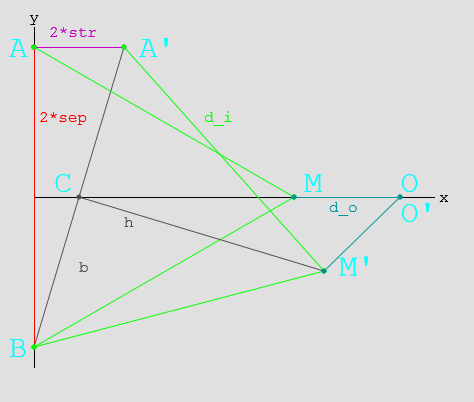Here, you can see the linkage layout for $$\{ false\ false \}$$ and $$\{ true\ false \}$$

# Mathematics

Ahead lurks tough mathematics (which may be unsuitable for liberal-arts majors)
If it’s hard to read, then sorry! It was hard to write!

## Parameters

• $$2 sep$$ Separation between inputs
• $$2 str$$ Stroke length $$false \to true$$
• $$d_i$$ Input link length
• $$d_o$$ Output link length

## Geometry

• $$A(x_a, y_a) = A(0, sep)$$ is the input position when $$A_{state}=false$$
• $$A'(x_a’, y_a’) = A'(2*str, sep)$$ is the input position when $$A_{state}=true$$
• $$B(x_b, y_b) = B(0,-sep)$$ is the input position (The system is symmetric, $$B’$$ does not need to be tested.)
• $$M(x_m, y_m)$$ is the middle position when $$A_{state}=false$$
• $$M'(x_m’, y_m’)$$ is the middle position when $$A_{state}=true$$
• $$O(x_o, y_o)$$ is the output position. ($$O \equiv O’$$ for the gate to function correctly.

## Solving

### Finding $$M$$:

$$M(x_m, y_m) = M( \sqrt{d_i^2 – sep^2}, 0)\\$$

### Finding $$O$$:

\begin{align} O(x_o, y_o) &= O( x_m + d_o, 0 )\\ & = O( \sqrt{d_i^2 – sep^2} + d_o, 0) \end{align}

### Finding $$M’$$:

• $$C'(x_c’, y_c’)$$ is the midpoint of $$BA’$$
• $$b$$ is the length of the line $$BC’$$
• $$h$$ is the length of the line $$C’M’$$
\begin{align} x_c’ &= (x_a’ + x_b)/2 \\ &= str\\ y_c’ &= (y_a’ + y_b)/2 \\ &= 0\\ b &= \sqrt{ sep^2 + str^2 }\\ h &= \sqrt{ d_i^2 – b^2 } && Because BC’ \bot C’M’\\ &= \sqrt{ d_i^2 – sep^2 – str^2 }\\ \end{align}\\

Using this, we can calculate M’:
\begin{align} \frac{h}{b} &= \frac{\sqrt{ d_i^2 – sep^2 – str^2 }}{\sqrt{ sep^2 + str^2 }} \\ &= \sqrt{\frac{ d_i^2 – sep^2 – str^2 }{ sep^2 + str^2 }} \\ &= \sqrt{\frac{d_i^2}{sep^2 + str^2} – 1}\\ \\ x_m’ &= x_c’\ – ( y_c’ – y_b)\frac{h}{b}\\ &= str – \sqrt{\frac{d_i^2}{sep^2 + str^2} – 1} \\ y_m’ &= y_c’ + ( x_c’ – x_b)\frac{h}{b}\\ &= str \sqrt{\frac{d_i^2}{sep^2 + str^2} – 1} \end{align}\\

### Finding $$O’$$:

\begin{align} x_o’ &= x_m’ + \sqrt{ d_o^2 – y_m’^2 }\\ &= ( str – \sqrt{\frac{ d_i^2 }{sep^2 + str^2} – 1}) + \sqrt{ d_o^2 – ( str \sqrt{\frac{d_i^2}{sep^2 + str^2} – 1} )^2 }\\ &= str – \sqrt{\frac{ d_i^2 }{sep^2 + str^2} – 1} + \sqrt{ d_o^2 – str^2 (\frac{d_i^2}{sep^2 + str^2} – 1) }\\ y_o’ &= 0 \end{align} \\

## And then:

Now that $$O$$ and $$O’$$ are found, and we know $$O \equiv O’$$…

### Finding the $$x_o’$$ equation in terms of $$d_o^2$$:

\begin{align} x_o’ &= x_m’ + \sqrt{ d_o^2 – y_m’^2 }\\ d_o^2 – y_m’^2 &= (x_o’ – x_m’)^2 \\ d_o^2 – str^2 (\frac{d_i^2}{sep^2 + str^2} – 1) &= (x_o’ – str + \sqrt{\frac{d_i^2}{sep^2 + str^2} – 1})^2 \\ d_o^2 &= \left( x_o’ – str + \sqrt{\frac{d_i^2}{sep^2 + str^2} – 1} \right)^2 + str^2 (\frac{d_i^2}{sep^2 + str^2} – 1) \\ \end{align}

### Substituting back into the $$x_o$$ equation:

\begin{align} x_o &= \sqrt{d_i^2 – sep^2} + d_o \\ x_o – \sqrt{d_i^2 – sep^2} &= d_o \\ ( x_o – \sqrt{d_i^2 – sep^2} )^2 &= d_o^2 \\ ( x_o – \sqrt{d_i^2 – sep^2} )^2 &= \left( x_o’ – str + \sqrt{\frac{d_i^2}{sep^2 + str^2} – 1} \right)^2 + str^2 (\frac{d_i^2}{sep^2 + str^2} – 1) \end{align}
Ugly, but workable?

# Example:

• $$sep = 50$$ Separation between inputs $$100 mm$$
• $$str = 20$$ Stroke length $$false \to true: 40 mm$$
• $$d_i = ?$$ Input link length
• $$d_o = ?$$ Output link length
• $$x_o = x_o’ = 70$$ Desired output position

\begin{align} ( x_o – \sqrt{d_i^2 – sep^2} )^2 &= \left( x_o’ – str + \sqrt{\frac{d_i^2}{sep^2 + str^2} – 1} \right)^2 + str^2 (\frac{d_i^2}{sep^2 + str^2} – 1) \\ ( 70 – \sqrt{d_i^2 – 50^2} )^2 &= \left( 70 – 20 + \sqrt{\frac{d_i^2}{50^2 + 20^2} – 1} \right)^2 + 20^2 (\frac{d_i^2}{50^2 + 20^2} – 1) \\ \end{align} \\
Pushed through Wolfram Alpha…
\begin{align} \\ d_i &= \sqrt{2900} \\ &= 53.852\\ \text{and}\\ d_o &= x_o – \sqrt{d_i^2 – sep^2} \\ &= 70 – \sqrt{ 2900 – 2500 } \\ &= 50 \end{align}

# Summary:

Phew!

Thusly, one can calculate the input and output linkage lengths for any logic gate.
These dimensions will work for an OR gate too, as the linkages are just reversed.

It’s irksome that I couldn’t work out a symbolic solution for the last equations, especially since $$d_i$$ in the example came out to such a neat value.

I might revisit this further in the future, and it’ll definitely come in handy when building something with these gates.

# Springless Reversible Mechanical Logic

Logic gates! The building blocks of computing as we know it. What if we ditch the electrons?

I want some mechanical logic for an upcoming project, so I did some research…

# Research

There are several examples of mechanical logic out there on the internet:

Keshav Saharia’s Lego LogicPush-pull, needs a spring return.
Randomwraith’s Lego LogicPush-pull with internal rotating elements, reversible.
Mechalogic’s Logic ElementsPush-pull, AND & NOT reversible, OR needs a spring return.
Xiaoji Chen’s Linkage ComputerRotating logic, reversible, some mechanical issues with parallelogram weakness.
Spillerrec’s Lego Logic GatesPush-pull, very compact, needs a spring return.
He identifies the problem with needing springs, but doesn’t have a fix. Mentions how AND/OR gates can be reversed to become OR/AND gates.
Zeroumus’s AND GatePush-pull, needs a spring return.
KNEX XOR GatePush-pull, needs a spring return.
Carolin Liebl and Lisa Hopf’s NAND GatePush-pull, reversible, complex.

There are many more ideas out there, even water pipe logic, and of course the early mechanical computers Digi-Comp I and Digi-Comp II.

For my project, I have the following requirements;

• Push-pull logic
• Output movement range must equal input movement range
• No springs/elastic bands, and no forces for inputs to overcome
• No gears!
• Easily chain-able, and simple enough to implement a score of them.

This disqualifies most of the designs I found, particularly the ‘no springs’ requirement.

# AND Gate

Mechalogic’s AND gate design uses an elastic band, but doesn’t appear to need it, so it serves as a good starting point: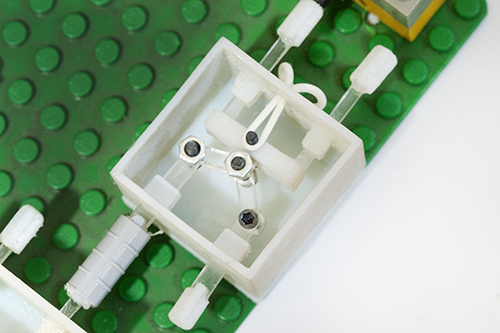It’s time consuming to tweak parameters in the physical world, so I wrote a small simulation: (click to see in action)Success! Meets all the requirements, and only needs three parts.

The geometry is important – the ratio will determine the output’s position when only one of the inputs is asserted, according to;

• distance between the inputs (red),
• length of the input links (green),
• length of the output link (teal)
• input displacement

Now, can an OR gate be designed with the same success?

# OR Gate

Most OR gates look like this; the output (on the left of this picture) has a flat plate, and either input will push the flat forward. Unfortunately the design needs that elastic band to return the output to zero.Inspired by some earlier attempts to couple an AND and a backwards OR gate together, I tried flipping the AND gate around; (click to see in action)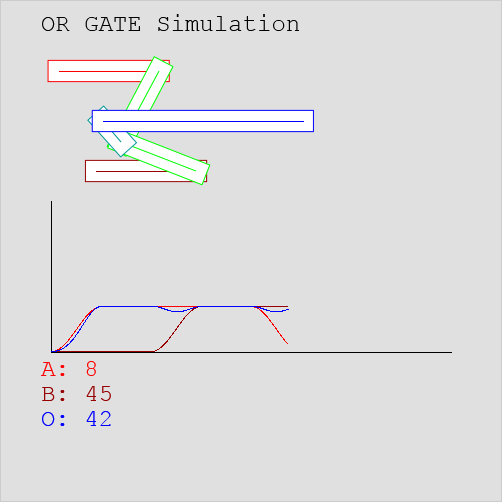Success! Oddly enough, an OR gate is just an AND gate connected up backwards.
Even the linkage lengths are the same!
Best of all, the OR gate is reversible like the AND gate – no springs and no force required.

# AND Then?

As the graphs show, output displacement equals input displacement, so these designs should be chainable. Let’s have a look; (click to see in action)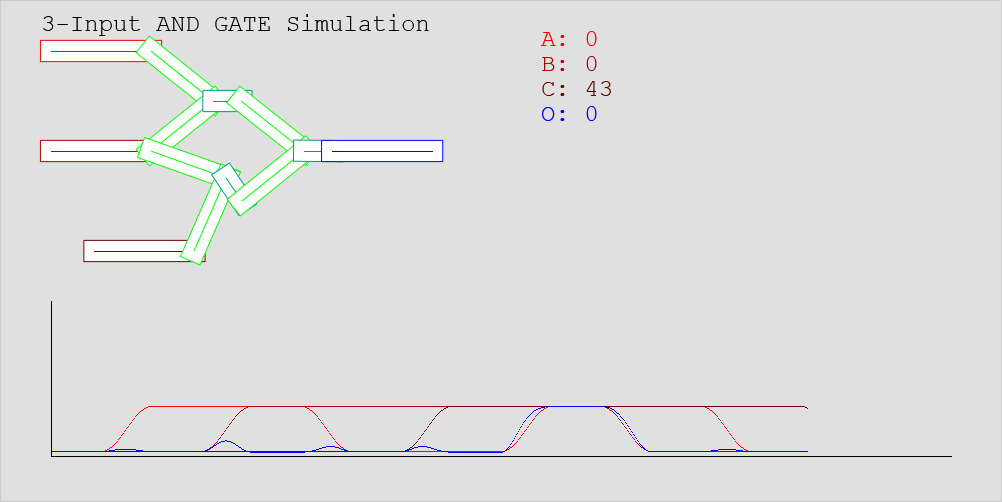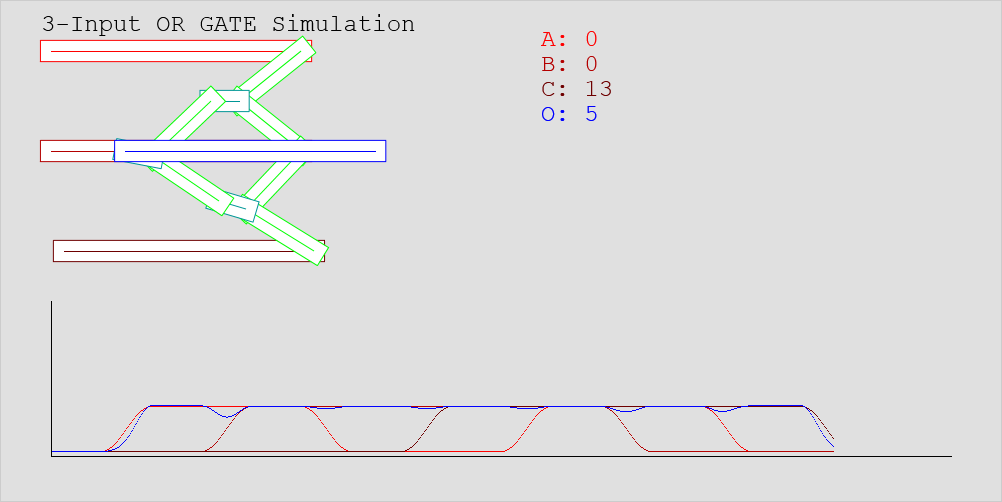Success!

Now I just have to build something complex with them…

All the simulations are viewable here.
The code they’re written with is available on GitHub;
https://github.com/MrTrick/logic_gates

# Eagle: Text Silkscreen Width

Thanks to Hackvana, I’m making teeny tiny circuit boards!
No matter how good a board house’s process, there are some fundamental limits to how small things a board and the design on it can be.

A PCB is much easier to understand with a silkscreen! However, you can’t draw silkscreen lines narrower than a certain width, often about 5 mils. (Or 4 mils if you’re really lucky) So you’d better make sure none of your silkscreen is smaller, or lines will be spotty and text will be unreadable.

(Huh? What’s a mil? / One thousandth of an inch. / Okay. What’s an inch? / …)

With Eagle able to display line widths in mils, it’s not too difficult to check your lines.
(How do I get mils? / Click on the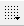Grid button in the upper left, and change the grid units to mils. You can change it back later)

Here is the text “ISP”, and the text attributes dialog.

• Note that font has been set to vector. Keep your text in vector mode, it will ensure that the Gerber files you output are likely to match what you see inside Eagle.
• The grid size in these examples has been set to 10 mils
• There are two important settings – Size and Ratio.

Size is the height of one row of text.
Ratio is the text’s line width, as a percentage of size.

• For the above image, the line width is 5% of 20mils – only 1mil! That definitely won’t work!
• Remember; SIZE x RATIO > 5 mils

## But I Want Small Text!

That’s fine. You’ll just need to make it thicker.

These are still a bit small…

This one is probably juuust thick enough. But the text is only half a millimetre high!

# Okay, I’ll be sensible…

Good. 50 mils (1.27mm) or larger text is probably more sensible. If size is 50, and we need at least 5 mil silkscreen, then the ratio should be higher than 10%. Easy!

# In Conclusion

A board house will often tell you their dimension limits, for traces, isolation, and silkscreen.
For best results, avoid the limits.

If you can make your text 10 mil thick, and the board house can manage down to 5 mil, you should have no problems.

# I2C and Device Limits

SparkFun’s Peter Dokter has an interesting video talking about SPI and i2c comms. i2c in particular is a great bus mechanism, due to the microprocessor only needing TWO wires to control (almost) any number of devices.

Each device on an i2c network has a 7-bit address, so a single network theoretically supports up to 128 slave devices.In practice though, the limit is much lower. i2c slave chips often support only 8 different bus addresses, no more than 8 of that chip can be attached to the same i2c network. Others like the MPL3115A2 aren’t configurable at all! Some people really do want more than one altimeter…

You could have more than one chip to an address, it just means that the chips change in lockstep with each other, and any returned values are likely to be garbage. (being ANDed together by the open-drain bus)

Enter the i2c PCA9547 multiplexer. It allows you to have multiple i2c ‘channels’, and decide which one is active at any one time. That way, you could for instance have one MPL3115A2 altimeter on each channel, for a total of 8 in your system instead of just one. Ironically, the multiplexer suffers from the same address issues as the other chips; You can fit only 8 multiplexers on an i2c bus.

Or you can be sneaky… What’s the maximum number of uniquely controllable 1-address i2c devices you can put on a multiplexed i2c network?

# Step 0: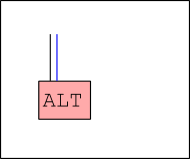One bus, one device. Not much fun.

# Step 1: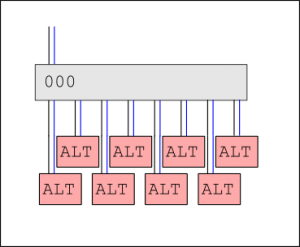Let’s add a PCA9547 multiplexer! Now we have 8 switchable channels, and 8 individually controllable devices.

But we can do better.

# Step 2: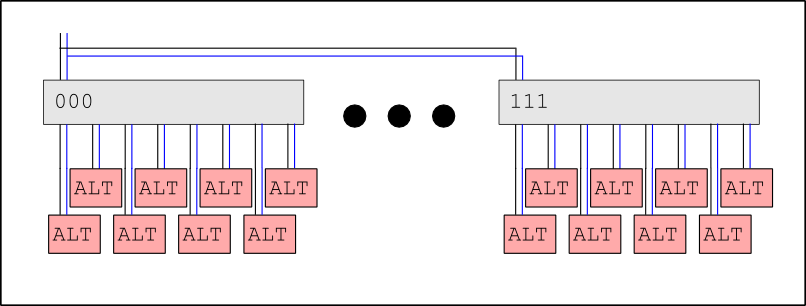Each multiplexer can be given one of 8 addresses in the space 1110xxx. In shorthand, we’ll call them 000 to 111. So, we can attach up to 8 multiplexers to any one bus.

8 multiplexers × 8 channels = 64 devices, that each get a whole bus channel to themselves!

We can do better.

# Step 3: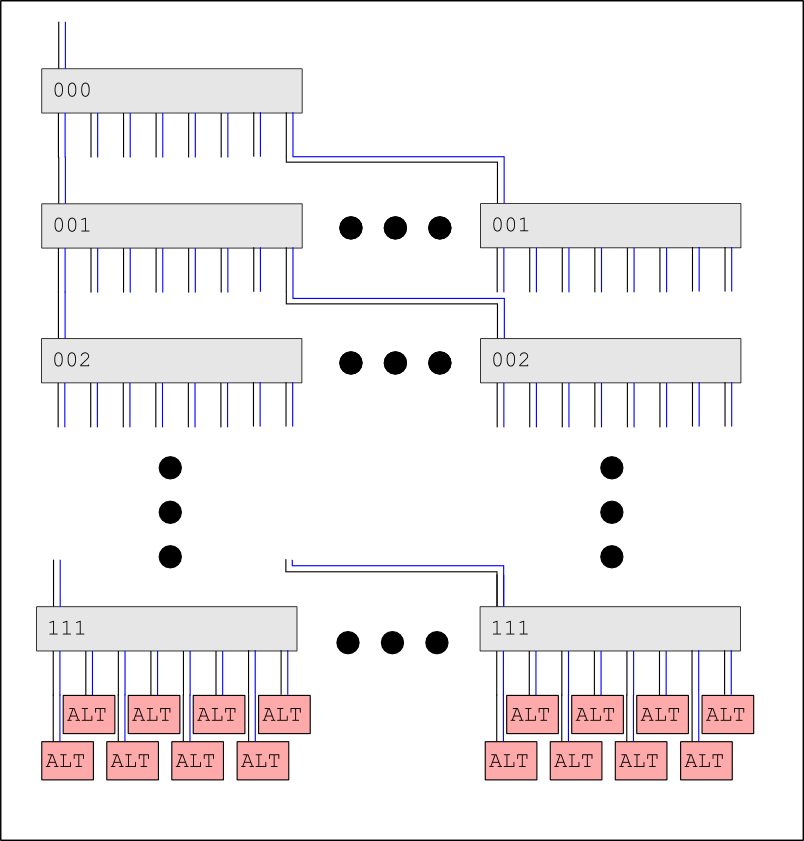Not only can we attach multiple multiplexers to one bus, we can attach a multiplexer to another multiplexer. In this simple tree configuration, the controller is connected to a multiplexer with address 000. Each of 000‘s 8 channels is connected to a multiplexer with address 001. (For the same reason that we can have multiple devices with the same address if they’re not simultaneously connected, we can put same-address multiplexers on different channels.)

So, going down the tree, there are:
1 of 000
8 of 001
64 of 010
… and so on, down to;
16,777,216 of 111
And each 111 multiplexer has 8 channels, so we have 134,217,728 individually selectable bus segments, and can support that many single-address devices. Should be enough?

At this scale, we’d need 19 MILLION multiplexers, which not counting the net routing, the pull-up resistors, the power requirements, or the devices we’re supporting, would require (according to the package size) a circuit board with an area of more than 300 square metres.

We can do better!

# Step 4: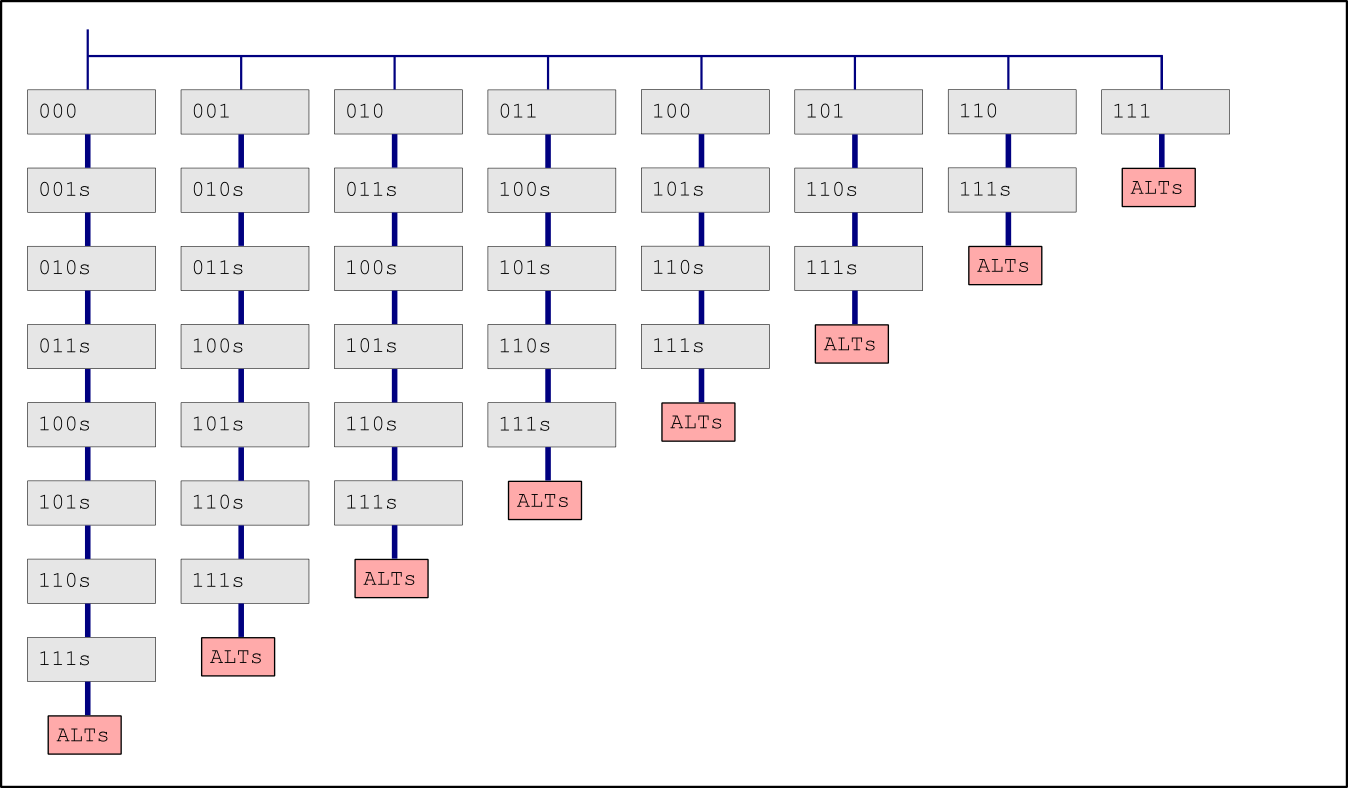(It becomes unwieldy at this stage to try and draw even collapsed collections of multiplexers, so for the above, imagine that boxes with plural labels indicate multiple chips, and thick connections represent the 1:8 fan-out discussed previously.)

Reading the PCA9547 datasheet, it mentions that you can also disable the multiplexer entirely so that no channels are selected. This allows another fun technique. In the above diagram, the left-most column is the tree structure show in the previous step. The second column is a slightly smaller tree starting at 001, and so-on.

By disabling all top-level multiplexers, then only enabling the multiplexers needed, all of the devices in all the trees can be accessed. The diagram only shows the first generation of extra multiplexers, this process can be recursively applied to each sub-tree.

Eg to access a channel in multiplexer 101 → 3 → 1 → 7:

1. Disable all multiplexers
2. Enable 101, select channel 3
3. Enable 110, select channel 1
4. Enable 111, select channel 7
(At this stage, multiple 110 and 111 multiplexers are ‘on’. We need to switch them off)
5. Disable 101
6. Disable 111
7. Disable 110
8. Re-enable 101. Now only the correct path is open, and the device can be accessed.

Diagram here

This adds 19 million extra devices on top of the 134 million we get from the simpler tree in the previous step. Now, that’s enough?

A few more can be added through further recursion, but there are diminishing returns.

# Step 5:

Left as an exercise for the reader. What if you have multiple i2c multiplexers with the same address in sequence? If initially active, sending ‘disable’ would probably switch all of them off. And then sending ‘enable’ would activate only the first one…